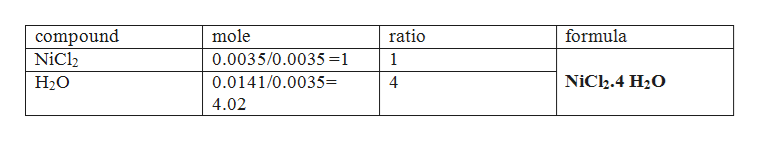# In lab, a student was given a hydrated version of NiCl2 as the unknown. The initial mass of the hydrated salt was 0.712 g. The student heated the crucible for 10 minutes with the cover slightly ajar. After cooling, the dried salt weighed 0.458 g. What is the chemical formula for this hydrate? What is the IUPAC name for this hydrate?

Question
16 views

In lab, a student was given a hydrated version of NiCl2 as the unknown. The initial mass of the hydrated salt was 0.712 g. The student heated the crucible for 10 minutes with the cover slightly ajar. After cooling, the dried salt weighed 0.458 g. What is the chemical formula for this hydrate? What is the IUPAC name for this hydrate?

check_circle

Step 1

During heating mass of the sample reduces due to loss of water. So, 0.458 g of sample remaining after drying will be the mass of salt NiCl2.

Step 2

Therefore number of moles of NiCl2 in 0.458 g of NiCl2 = given mass / molecular mass = 0.458 g/ 129.59 g/mol =0.0035 mol.

Mass of the water initially present in the sample = 0.712g -0.458...help_outlineImage Transcriptionclosecompound NiCl2 mole ratio formula 0.0035/0.0035 =1 1 NiCh.4 H20 0.0141/0.0035= Н20 4 4.02 fullscreen

### Want to see the full answer?

See Solution

#### Want to see this answer and more?

Solutions are written by subject experts who are available 24/7. Questions are typically answered within 1 hour.*

See Solution
*Response times may vary by subject and question.
Tagged in

### General Chemistry